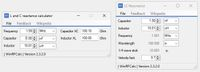# Reactance and resonance calculators [V2.3.2.0]

With these two calculators you can calculated the reactive impedance's of individual L and C components and the resonance frequency when an inductor and capacitor are combined in a resonant series or parallel circuit.

In the examples below it is demonstrated  that equal reactances have a resonance frequency and vise versa.

Additionally, if you need a 1/4 wave stub transformer the coaxial length based on frequency and cable velocity factor is determined.>100 RF Calculators
WinRFCalc, the best RF calculator toolbox for Windows